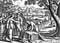Dido Purchases Land for the Foundation of Carthage, Matthäus Merian the Elder, 1630. Wikimedia Commons

# Calculus and the Legend of the Founding of Carthage

The ancient Romans had a story about the foundation of the ancient city of Carthage. According to Virgil’s epic poem the , Dido, the queen of the Phoenician city of Tyre, was forced to flee with her life when her brother usurped the throne and murdered her husband, the king. She eventually arrived in Libya, where she bargained with the king of the local tribes to be given as much land as could be enclosed by the hide of an ox. This may sound like a bad deal, but Dido was clever: She cut the hide into very thin strips…

# The Method of Lagrange Multipliers

Optimization, one of the elementary problems of mathematical physics, economics, engineering, and many other areas of applied math, is the problem of finding the maximum or minimum value of a function, called the objective function, as well as the values of the input variables where that optimum value occurs. In elementary single-variable calculus, this is a very simple problem that amounts to finding the values of for which d/d is zero.

Multivariable optimization problems are more complicated because we can impose constraints. We consider constraints that can be expressed in the form ()= for some function and constant…

# Why Trisecting the Angle is Impossible

Suppose that two lines L₁ and L₂ meet at point P and that the angle θ between the lines is arbitrary. One of the elementary problems in classical geometry is to bisect the angle, that is, to construct a line through P which makes an angle of θ/2 with L₁ and L₂ using only a compass (a tool which can draw a perfect circle of any radius centered at a point) and an straightedge (a tool which can draw a perfectly straight line through any two points but which cannot be used as a ruler to measure lengths).

# Laplace’s Equation

Having looked at the heat equation and the wave equation, we now come to the third of the most common partial differential equations in physics, Laplace’s equation:

# Thinking Relativistically

In my last article on special relativity, I explained how and why our intuitive understanding of space and time needed to be modified to account for developments in electromagnetic theory that took place in the 19th century, in particular the results of the Michelson-Morley experiment and the non-covariance of the electromagnetic wave equation under the Galilean transform. …

# Kepler’s Laws of Planetary Motion

Between 1609 and 1619, Johannes Kepler used data collected by Tycho Brahe to deduce the laws that determine the motion of the planets around the sun:

• Every planet moves in an elliptical orbit with the Sun at one of the foci.
• The planet moves in its orbit, a line drawn from the Sun to the planet will sweep out equal areas in equal times.
• The square of the period of the orbit is proportional to the length of semi-major axis of the elliptical orbit.

These three laws are now named Kepler’s laws in his honor.

This was a surprise to…

# Schrödinger’s Equation

Last time, we discussed the dual nature of matter, in which particles behave like waves and waves behave like particles. To explain this we introduce the wave function, which describes not the actual position of a particle, but the probability of finding the particle at a given point. Furthermore, when we consider the wave function as describing the state of a “probability field”, we see that the time-dependent behavior of this field exhibits wave-like behavior.

Suppose that the particle’s interactions with the world are represented by a potential energy function V(r) which We will…

# Root-Finding Algorithms

## Ways of determining when an equation is equal to zero

As every schoolchild knows, the solutions of a quadratic polynomial equation ax²+bx+c=0 are given by the quadratic formula:

But in the real world, we don’t just have to deal with second degree polynomials. Finding the roots of higher-order polynomials, or roots of transcendental equations like x-arctan(x)=0, can be a challenge because:

• While there are formulas analogous to the quadratic formula for third and fourth degree polynomials, they are very complicated and aren’t generally worth using.
• There is no general formula that gives the roots of polynomials of degree five and greater, by the Abel-Ruffini theorem.
• Transcendental equations do not generally…

# The Arithmetic-Geometric Mean Inequality

Suppose that and are non-negative real numbers, not necessarily distinct. The famous arithmetic-geometric mean inequality says that:

With equality if and only if . This generalizes to the case of non-negative numbers:

# What is Wave-Particle Duality?

One of the most well-known ideas in quantum physics, and one of the ideas most frequently held up as an example of the mysterious nature of quantum physics, is that material objects exist simultaneously as particles and as waves. Material objects exist as particles in the sense that they can be assigned definite quantities like location and momentum, but they also exist as waves in the sense that they can be described as a propagating disturbance in some underlying (possibly very abstract) field.